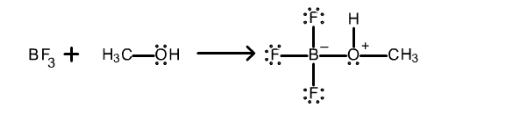# Problem: For the following reaction, indicate which reactant is the Lewis acid and which is the Lewis base.a. BF3 is the Lewis acid.b. BF3 is the Lewis base.c. CH3OH is the Lewis acid.d. CH3OH is the Lewis base.e. This not a Lewis acid-base reaction.

🤓 Based on our data, we think this question is relevant for Professor Luzik's class at University of New Haven.

###### Problem Details

For the following reaction, indicate which reactant is the Lewis acid and which is the Lewis base.

a. BF3 is the Lewis acid.

b. BF3 is the Lewis base.

c. CH3OH is the Lewis acid.

d. CH3OH is the Lewis base.

e. This not a Lewis acid-base reaction.What scientific concept do you need to know in order to solve this problem?

Our tutors have indicated that to solve this problem you will need to apply the Lewis Acid and Base concept. You can view video lessons to learn Lewis Acid and Base. Or if you need more Lewis Acid and Base practice, you can also practice Lewis Acid and Base practice problems.

What is the difficulty of this problem?

Our tutors rated the difficulty ofFor the following reaction, indicate which reactant is the L...as medium difficulty.

How long does this problem take to solve?

Our expert Chemistry tutor, Dasha took 2 minutes and 53 seconds to solve this problem. You can follow their steps in the video explanation above.

What professor is this problem relevant for?

Based on our data, we think this problem is relevant for Professor Luzik's class at University of New Haven.